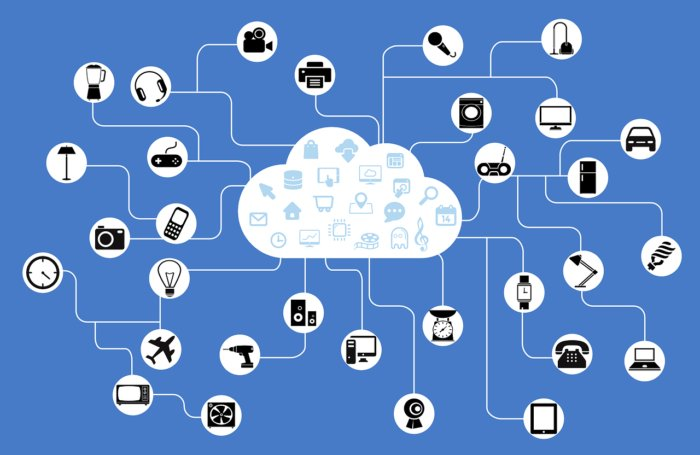## Applied Category Theory Talks at QPL2019Here are some talks on applied category theory:

#### John Baez, Structured cospans

Open systems of many kinds can be treated as morphisms in symmetric monoidal categories. Two complementary approaches can be used to work with such categories: props (which are more algebraic in flavor) and cospan categories (which are more geometrical). In this talk we focus on the latter. Brendan Fong’s “decorated cospans” are a powerful tool for treating open systems as cospans equipped with extra structure. Recently Kenny Courser has found a simpler alternative, the theory of “structured cospans”. We describe this theory and sketch how it has been applied to a variety of open systems, such as electrical circuits, Markov processes, chemical reactions and Petri nets.

You can see the slides here. For more on structured cospans, go here:

#### Kenny Courser, Coarse-graining open Markov processes

Coarse-graining is a standard method of extracting a simpler Markov process from a more complicated one by identifying states. Here we extend coarse-graining to 'open' Markov processes: that is, those where probability can flow in or out of certain states called 'inputs' and 'outputs'. One can build up an ordinary Markov process from smaller open pieces in two basic ways: composition, where we identify the outputs of one open Markov process with the inputs of another, and tensoring, where we set two open Markov processes side by side. In previous work, Fong, Pollard and the first author showed that these constructions make open Markov processes into the morphisms of a symmetric monoidal category. Here we go further by constructing a symmetric monoidal double category where the 2-morphisms include ways of coarse-graining open Markov processes. We also extend the already known 'black-boxing' functor from the category of open Markov processes to our double category. Black-boxing sends any open Markov process to the linear relation between input and output data that holds in steady states, including nonequilibrium steady states where there is a nonzero flow of probability through the process. To extend black-boxing to a functor between double categories, we need to prove that black-boxing is compatible with coarse-graining.
You can see the slides here. For more on coarse-graining open Markov processes, go here:

#### Jade Master, Open Petri nets

The reachability semantics for Petri nets can be studied using open Petri nets. For us an 'open' Petri net is one with certain places designated as inputs and outputs via a cospan of sets. We can compose open Petri nets by gluing the outputs of one to the inputs of another. Open Petri nets can be treated as morphisms of a category $$\mathsf{Open}(\mathsf{Petri})$$, which becomes symmetric monoidal under disjoint union. However, since the composite of open Petri nets is defined only up to isomorphism, it is better to treat them as morphisms of a symmetric monoidal double category $$\mathbb{O}\mathbf{pen}(\mathsf{Petri})$$. Various choices of semantics for open Petri nets can be described using symmetric monoidal double functors out of $$\mathbb{O}\mathbf{pen}(\mathsf{Petri})$$. Here we describe the reachability semantics, which assigns to each open Petri net the relation saying which markings of the outputs can be obtained from a given marking of the inputs via a sequence of transitions. We show this semantics gives a symmetric monoidal lax double functor from $$\mathbb{O}\mathbf{pen}(\mathsf{Petri})$$ to the double category of relations. A key step in the proof is to treat Petri nets as presentations of symmetric monoidal categories; for this we use the work of Meseguer, Montanari, Sassone and others.
You can see the slides here. For more, see this paper:

#### Joe Moeller, Network Models from Petri Nets with Catalysts

Petri networks and network models are two frameworks for the compositional design of systems of interacting entities. Here we show how to combine them using the concept of a 'catalyst': an entity that is neither destroyed nor created by any process it engages in. In a Petri net, a place is a catalyst if its in-degree equals its out-degree for every transition. We show how a Petri net with a chosen set of catalysts gives a network model. This network model maps any list of catalysts from the chosen set to the category whose morphisms are all the processes enabled by this list of catalysts. Applying the Grothendieck construction, we obtain a category fibered over the category whose objects are lists of catalysts. This category has as morphisms all processes enabled by some list of catalysts. While this category has a symmetric monoidal structure that describes doing processes in parallel, its fibers also have non-symmetric monoidal structures that describe doing one process and then another while reusing the catalysts.
You can see the slides here. For more, see this paper: